Test: Fractions- 1

# Test: Fractions- 1

Test Description

## 10 Questions MCQ Test Mathematics for Class 5 | Test: Fractions- 1

Test: Fractions- 1 for Class 5 2023 is part of Mathematics for Class 5 preparation. The Test: Fractions- 1 questions and answers have been prepared according to the Class 5 exam syllabus.The Test: Fractions- 1 MCQs are made for Class 5 2023 Exam. Find important definitions, questions, notes, meanings, examples, exercises, MCQs and online tests for Test: Fractions- 1 below.
Solutions of Test: Fractions- 1 questions in English are available as part of our Mathematics for Class 5 for Class 5 & Test: Fractions- 1 solutions in Hindi for Mathematics for Class 5 course. Download more important topics, notes, lectures and mock test series for Class 5 Exam by signing up for free. Attempt Test: Fractions- 1 | 10 questions in 15 minutes | Mock test for Class 5 preparation | Free important questions MCQ to study Mathematics for Class 5 for Class 5 Exam | Download free PDF with solutions
 1 Crore+ students have signed up on EduRev. Have you?
Test: Fractions- 1 - Question 1

### Find an equivalent fraction of 14 / 35.

Detailed Solution for Test: Fractions- 1 - Question 1

Equivalent fraction of a given fraction is obtained by multiplying its numerator and denominator by the same number.

Test: Fractions- 1 - Question 2

### Observe the fractions given in the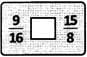Which symbol must be placed in the box to make the statement correct?

Detailed Solution for Test: Fractions- 1 - Question 2

On cross-multiplication, we get 9 × 8 ×__ 15 × 16
72 < 240
9 / 16 < 15 / 8.

Test: Fractions- 1 - Question 3

### Which of the following improper fractions is equivalent to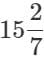?

Detailed Solution for Test: Fractions- 1 - Question 3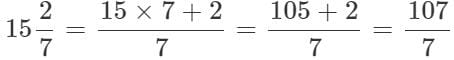Test: Fractions- 1 - Question 4

Which mixed number is equal to 45 / 14?

Detailed Solution for Test: Fractions- 1 - Question 4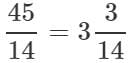Test: Fractions- 1 - Question 5

What is the sum of 5 / 13, 11 / 13 and 13 / 13?

Detailed Solution for Test: Fractions- 1 - Question 5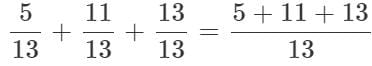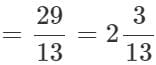Test: Fractions- 1 - Question 6

By what number is 19 / 25 lesser than 27 / 25?

Detailed Solution for Test: Fractions- 1 - Question 6

The required number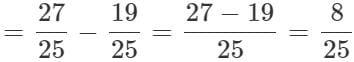Test: Fractions- 1 - Question 7

Find the difference obtained when 9 / 40 is subtracted from 23 / 40.

Detailed Solution for Test: Fractions- 1 - Question 7

The required difference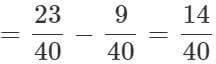Test: Fractions- 1 - Question 8

Which of the following is a proper fraction?

Detailed Solution for Test: Fractions- 1 - Question 8

The numerator of a proper fraction is less than its denominator.

Test: Fractions- 1 - Question 9

How is five-eighteenths written?

Detailed Solution for Test: Fractions- 1 - Question 9

Five - eighteenth = 5 / 18.

Test: Fractions- 1 - Question 10

Ravi had 5 / 6 of a cake. He ate 2 / 3 of it. What part of the cake is remaining?

Detailed Solution for Test: Fractions- 1 - Question 10

Part of the cake Ravi had = 5 / 6
Part of the Ravi's cake eaten = 2 / 3
∴ Part of the cake eaten = 2 / 3 × 5 / 6 = 5 / 9
∴ Remaining part of the cake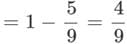## Mathematics for Class 5

12 videos|55 docs|35 tests
 Use Code STAYHOME200 and get INR 200 additional OFF Use Coupon Code
Information about Test: Fractions- 1 Page
In this test you can find the Exam questions for Test: Fractions- 1 solved & explained in the simplest way possible. Besides giving Questions and answers for Test: Fractions- 1, EduRev gives you an ample number of Online tests for practice

## Mathematics for Class 5

12 videos|55 docs|35 tests